The COUNTREG Procedure

Variable Selection

Subsections:

Variable Selection Using an Information Criterion

This type of variable selection uses either Akaike’s information criterion (AIC) or the Schwartz Bayesian criterion (SBC) and either a forward selection method or a backward elimination method.

Forward selection starts from a small subset of variables. In each step, the variable that gives the largest decrease in the value of the information criterion specified in the CRITER= option (AIC or SBC) is added. The process stops when the next candidate to be added does not reduce the value of the information criterion by more than the amount specified in the LSTOP= option in the MODEL statement.

Backward elimination starts from a larger subset of variables. In each step, one variable is dropped based on the information criterion chosen.

You can force a variable to be retained in the variable selection process by adding a RETAIN list to the SELECT=INFO (or SELECTVAR=) option in your model. For example, suppose you add a RETAIN list to the SELECT=INFO option in your model as follows:

   MODEL Art = Mar Kid5 Phd  / dist=negbin(p=2) SELECT=INFO(lstop=0.001 RETAIN(Phd));


Then this causes the variable selection process to consider only those models that contain Phd as a regressor. As a result, you are guaranteed that Phd will appear as one of the regressor variables in whatever model the variable selection process produces. The model that results is the "best" (relative to your selection criterion) of all the possible models that contain Phd.

When a ZEROMODEL statement is used in conjunction with a MODEL statement, then all the variables that appear in the ZEROMODEL statement are retained by default unless the ZEROMODEL statement itself contains a SELECT=INFO option.

For example, suppose you have the following:

   MODEL Art = Mar Kid5 Phd  / dist=negbin(p=2) SELECT=INFO(lstop=0.001 RETAIN(Phd));
ZEROMODEL Art ~ Fem Ment / link=normal;


Then Phd is retained in the MODEL statement and all the variables in the ZEROMODEL statement (Fem and Ment) are retained as well. You can add an empty SELECT=INFO clause to the ZEROMODEL statement to indicate that all the variables in that statement are eligible for elimination (that is, need not be retained) during variable selection. For example:

  MODEL Art = Mar Kid5 Phd  / dist=negbin(p=2) SELECT=INFO(lstop=0.001 RETAIN(Phd));
ZEROMODEL Art ~ Fem Ment / link=normal SELECT=INFO();


In this example, only Phd from the MODEL statement is guaranteed to be retained. All the other variables in the MODEL statement and all the variables in the ZEROMODEL statement are eligible for elimination.

Similarly, if your ZEROMODEL statement contains a SELECT=INFO option but your MODEL statement does not, then all the variables in the MODEL statement are retained, whereas only those variables listed in the RETAIN() list of the SELECT=INFO option for your ZEROMODEL statement are retained. For example:

   MODEL Art = Mar Kid5 Phd  / dist=negbin(p=2) ;
ZEROMODEL Art ~ Fem Ment / link=normal SELECT=INFO(RETAIN(Ment));


Here, all the variables in the MODEL statement (Mar Kid5 Phd) are retained, but only the Ment variable in the ZEROMODEL statement is retained.

Variable Selection Using Penalized Likelihood

Variable selection in the linear regression context can be achieved by adding some form of penalty on the regression coefficients. One particular such form is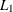norm penalty, which leads to LASSO: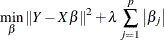This penalty method is becoming more popular in linear regression, because of the computational development in the recent years. However, how to generalize the penalty method for variable selection to the more general statistical models is not trivial. Some work has been done for the generalized linear models, in the sense that the likelihood depends on the data through a linear combination of the parameters and the data: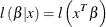In the more general form, the likelihood as a function of the parameters can be denoted by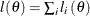, where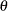is a vector that can include any parameters and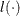is the likelihood for each observation. For example, in the Poisson model,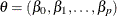, and in the negative binomial model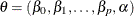. The following discussion introduces the penalty method, using the Poisson model as an example, but it applies similarly to the negative binomial model. The penalized likelihood function takes the form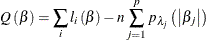The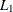norm penalty function that is used in the calculation is specified as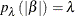The main challenge for this penalized likelihood method is on the computation side. The penalty function is nondifferentiable at zero, posing a computational problem for the optimization. To get around this nondifferentiability problem, Fan and Li (2001) suggested a local quadratic approximation for the penalty function. However, it was later found that the numerical performance is not satisfactory in a few respects. Zou and Li (2008) proposed local linear approximation (LLA) to solve the problem numerically. The algorithm replaces the penalty function with a linear approximation around a fixed point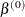: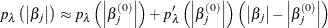Then the problem can be solved iteratively. Start from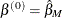, which denotes the usual MLE estimate. For iteration k,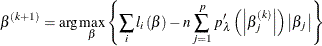The algorithm stops when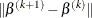is small. To save computing time, you can also choose a maximum number of iterations. This number can be specified by the LLASTEPS= option.

The objective function is nondifferentiable. The optimization problem can be solved using an optimization methods with constraints, by a variable transformation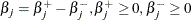For each fixed tuning parameter, you can solve the preceding optimization problem to obtain an estimate for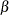. Because of the property of thenorm penalty, some of the coefficients incan be exactly zero. The remaining question is to choose the best tuning parameter. You can use either of the approaches that are described in the following subsections.

The GCV Approach

In the GCV approach, the generalized cross validation criteria (GCV) is computed for each value ofon a predetermined grid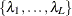; the value ofthat achieves the minimum of the GCV is the optimal tuning parameter. The maximum value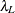can be determined by lemma 1 in Park and Hastie (2007) as follows. Suppose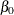is free of penalty in the objective function. Let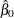be the MLE ofby forcing the rest of the parameters to be zero. Then the maximum value ofis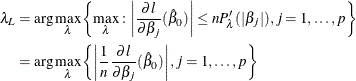You can compute the GCV by using the LASSO framework. In the last step of Newton-Raphson approximation, you have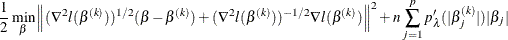The solution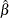satisfies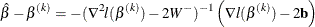where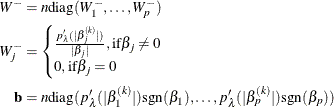Note that the intercept term has no penalty on its absolute value, and therefore the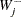term that corresponds to the intercept is 0. More generally, you can make any parameter (such as thein the negative binomial model) in the likelihood function free of penalty, and you treat them the same as the intercept.

The effective number of parameters is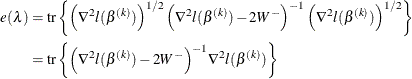and the generalized cross validation error is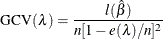The GCV1 Approach

Another form of GCV uses the number of nonzero coefficients as the degrees of freedom: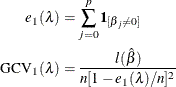The standard errors follow the sandwich formula: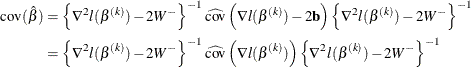It is common practice to report only the standard errors of the nonzero parameters.